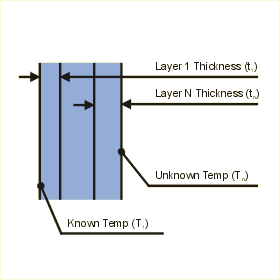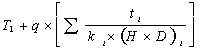Close

This page calculates the temperature on one side of an isothermal (constant temperature) multilayered plate or wall. You must define the heat load (Q) conducted through the layers as well as the temperature on one side of the plate (T1) .The temperature on the opposite side of the plate is calculated. For each layer you must define the layer thickness (ti) as well as the layer conductivity ki.The temperature (Tn) is calculated as:

Tn =Where T1 is the known surface temperature, Q is the heat flow (positive heat flow is from Tn to T1), ti is the thickness of the layer i,, ki is the thermal conductivity of layer i, H is the height of the plate, and D is the depth of the plate. The end surfaces of the plate are assumed adiabatic.

Positive values of heat (Q) result in Tn being hotter than T1. Negative values of heat (Q) result in Tn being cooler than T1.

References

Holman, J.P., Heat Transfer, 7th ed., McGraw Hill Book Company, New York, 1990, p 27-29.